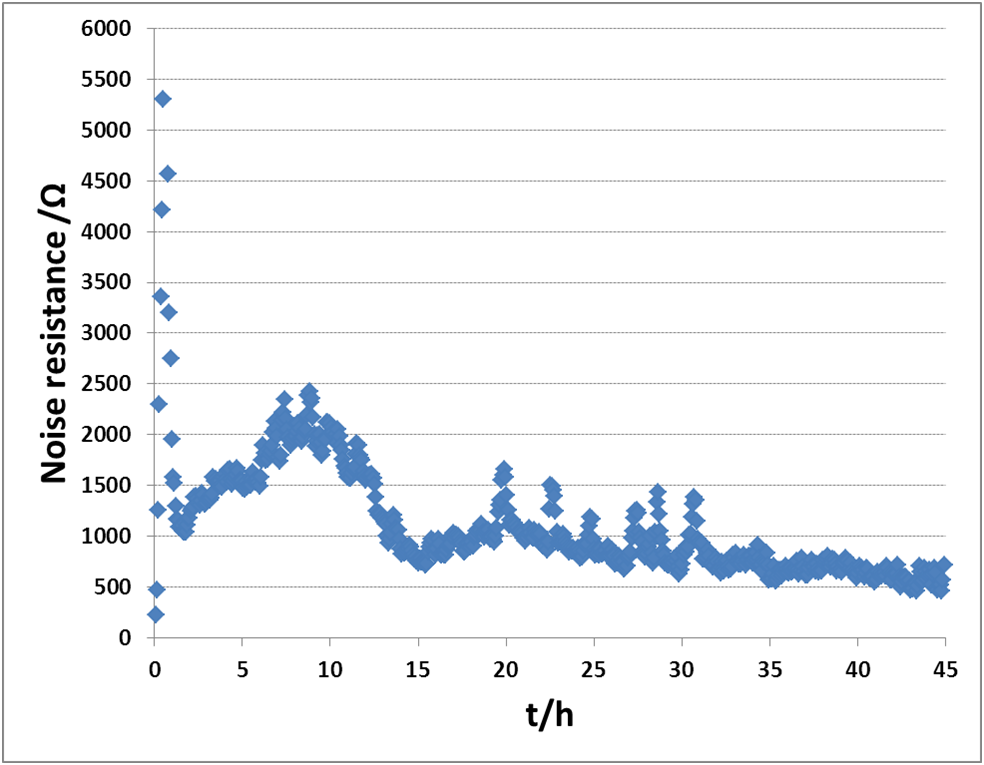11 min read# Electrochemical Noise Measurements: Part II: ASTM assessment on a real electrochemical system Corrosion –Application Note 39-2

Latest updated: September 15, 2023

Abstract

Noise measurements consist of recording the voltage and current fluctuations occurring when two electrodes of the same material (or not) are shorted. These measurements can lead to the determination of corrosion parameters. The fluctuations are of very small amplitude and this is why the choice of the instrument used in this purpose is critical. Efforts have been made by the American Society for Testing and Materials (ASTM) to give a procedure to characterize the measurement ability of the instrument. This second procedure involves a real electrochemical system namely a steel sample in acidic medium. A comparison of the noise resistance obtained using BioLogic instruments and by the authors lead to conclude that BioLogic instruments are suitable for noise measurements.

## Introduction

The conclusions of the part I were that the intrinsic potential and current noise of the BioLogic potentiostats are better than what is required by ASTM to perform valid noise measurements.

The objective of this, the second part of this application note is to take a step further in the validation of our instrument by comparing actual noise resistance measurements performed using BioLogic instruments to the results showed in the ASTM publication  on a real electrochemical system. Noise resistance is one of the many tools or values that can be used to interpret electrochemical noise data. It is written Rn and is generally defined as the standard deviation of the potential, divided by the standard deviation of the current :

$$R_n=\frac{\sigma_E}{\sigma_I} \tag{1}$$

## ASTM Experiment

The test system is AISI 1018 steel in 0.1 M citric acid. The exact conditions used are described in more details in the ASTM publication  as well as in ASTM G5 . In our experiment, we used a corrosion cell EL-CORR-1 along with three sample holders 092-C-013, in which one AISI 1018 disk for each holder was inserted. The same material was used for the working, the counter, and the pseudo-reference electrode. As the three electrodes formed an equilateral triangle, each electrode plane was equidistant from one another. Each sample was polished with a SiC paper at 240 and 600 grit, rinsed in ethanol and dried in air. The ambient temperature was kept at 25 °C and the solution was left in open air with no additional stirring. The cell was placed in a Faraday Cage and a VSP-300 was used to perform the measurement.

EC-Lab®  10.20 and the ZRA technique were used. The conditions are shown in Fig. 1. The default filter (50 kHz) was set

## ASTM Comparison

Figure 2 shows the evolution of the Rn obtained in the ASTM publication. Rn was defined as follows :

$$R_n=\frac{E_{rms}}{I_{rms}} \tag{2}$$

where Erms and Irms are the Root Mean Square values of the potential and current noise, respectively, recorded during a period of 256 s. See Appendix for the definition of the rms values.

######Figure 3: Electrochemical noise resistance for AISI 1018 steel in 0.1 M citric acid obtained using a VSP-300.

Figure 3 shows the results obtained in similar conditions using a VSP-300. By comparing Fig. 2 and Fig. 3, it can be concluded that the noise measurements performed using BioLogic’s VSP-300 yielded results that were very similar to those obtained in the ASTM publication and used as a reference. After 40 h, Rn reached a value of 500 Ω in our case and was somewhere between 1,000 and 2,000 Ω, in the ASTM publication. These discrepancies could be due to the slight differences in the experimental set-up (aeration, temperature, purity of the products etc ). Mass loss measurements were performed on the working electrode. Assuming for the working electrode, a density of 7.8 g cm-3 and a total exposure duration of 73.4 h, the corrosion rate was found to be close to 1 mm/y, which corresponds to the values given in the ASTM publication .

## Conclusion

According to the results and the recommendations provided by ASTM, BioLogic instruments are capable of performing reliable noise measurements on real electrochemical systems.

Data files can be found in :

C:\Users\xxx\Documents\EC-Lab\Data\Samples\Corrosion\AN_39_ZRA_II_XXX

## Appendix

The standard deviation of a distributed value x is:

$$\sigma\left(x\right)=\sqrt{\frac{1}{n-1}\sum_{i}\left(x_i-\mu\left(x\right)\right)^2} \tag{3}$$

where n is the number of the value x and μ(x) the mean value.

The rms value is defined as:

$$rms\left(x\right)=\sqrt{\frac{1}{n}\sum_{i}\ x_i^2} \tag{4}$$

If n is very large and µ(x) = 0 then:

rms(x) = σ(x).

## References

1) J.R. Kearns, J.R. Scully, “Electrochemical Noise Measurements for Corrosion Applications”, ASTM Intl, West Conshohocken, (1996) 446.
2) R. A. Cottis, Corrosion 57, 3 (2001) 265.
3) ASTM G5-94 Standard Reference Test Method for Making Potentiostatic and Potentiodynamic Anodic Polarization Measurements.

Revised in 08/2019

Work smarter. Not harder.

Tech-tips, theory, latest functionality, new products & more.

No thanks!